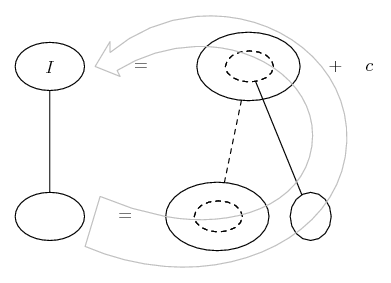# Thread: Finding volume by using triple integral

1. ## Finding volume by using triple integral

I need to find the volume enclosed by $\displaystyle x^2+y^2+z^2=a^2$ and $\displaystyle x^2+y^2=ax$ where $\displaystyle a>0.$

How do I find the bounds? Do I apply spherical coordinates as written?

2. To start with, I expect that you have written the second bound wrongly, since that is the equation of a plane figure, not a solid...

3. I edited the first equation, but what's wrong with the second one?

4. It's a 2 Dimensional object, i.e. a plane figure. How are we supposed to know where along the $\displaystyle \displaystyle z$ axis it's supposed to lie?

5. Assuming the cylinder extends indefinitely up and down the z dimension, we have Viviani's Curve. Doing just the top half, we have z going from 0 (where it 'starts', on the (x,y) plane) up to $\displaystyle \sqrt{a^2 - r^2}$ (where it hits the hemisphere). And we have r going from 0 at the centre (z axis), up to a cos theta i.e. everywhere inside the cylinder. And theta is turning through the x-positive half of the space, i.e. from minus pi/2 to pi/2. So...

$\displaystyle \displaystyle{V = \int_{-\frac{\pi}{2}}^{\frac{\pi}{2}}\ \int_{0}^{a \cos \theta} \int_{0}^{\sqrt{a^2 - r^2}} r\ dz\ dr\ d\theta}$

Just in case a picture helps to follow through from the inside out, we can start bottom left here, integrating r with respect to z...... where (key in spoiler) ...

Spoiler:... is the chain rule. Straight continuous lines differentiate downwards (integrate up) with respect to the main variable (in this case r), and the straight dashed line similarly but with respect to the dashed balloon expression (the inner function of the composite which is subject to the chain rule).

The general drift is...Which leaves a couple of blanks to fill. Hope this helps.

_________________________________________

Don't integrate - balloontegrate!

Balloon Calculus; standard integrals, derivatives and methods

Balloon Calculus Drawing with LaTeX and Asymptote!

6.Originally Posted by Prove ItTo start with, I expect that you have written the second bound wrongly, since that is the equation of a plane figure, not a solid...
No, it's not.$\displaystyle x^2+ y^2= ax$ where z can be anything is a cylinder. Specifically, it is the cylinder with central axis (a/2, 0, z) and radius a/2.

7.Originally Posted by HallsofIvyNo, it's not.$\displaystyle x^2+ y^2= ax$ where z can be anything is a cylinder. Specifically, it is the cylinder with central axis (a/2, 0, z) and radius a/2.
Then it should have been stated that $\displaystyle \displaystyle z$ can be anything...

8. No, it was stated that this problem was in three dimensions. The fact that there was no restriction put on z meant that it could be anything.

,

### volume between sphere and cylinder viviani

Click on a term to search for related topics.

#### Search Tags

finding, integral, triple, volume